## Example Questions

### Example Question #111 : Integers

Assumeand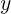are both even whole numbers and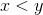.

What is a possible solution of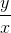?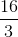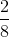Explanation:

Since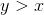, then the final answer will be a number greater than on top as well as an even number since bothandare even whole numbers. The only answer that fits both requirements is.

### Example Question #112 : Integers

Choose the answer below which best solves the following equation: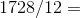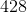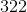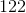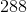Explanation: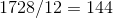To solve this problem, set up long division for yourself.  First, you know that your hundreds digit of the solution will be one, as twelve goes into seventeen one time.  Then, you take twelve away from seventeen, and are left with three remaining.  Bring down your next digit over from the dividend, and you are left with thirty two, which twelve goes into four times.  Already, you know that the answer has to beas none of the other answers fit the correct pattern.

### Example Question #113 : Integers

Solve for: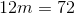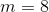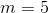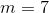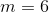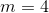Explanation:

To solve, first isolate the variable by dividing both sides of the equation by: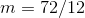As a check, know that any time you divide an even number by another even number, you will get an even result.

### Example Question #114 : Integers

Solve for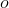: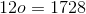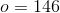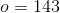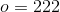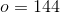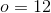Explanation:

To solve, isolate your variable by dividing both sides of the equation by: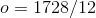As a check, know that any time you divide an even number by another even number, the result will be even.

### Example Question #115 : Integers

Solve for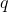: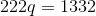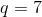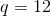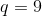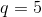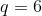Explanation:

To solve, isolate the variable by dividing both sides of the equation by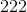: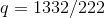As a check, know that any time you divide an even number by another even number, the result will be even.

### Example Question #116 : Integers

Solve for: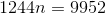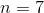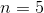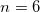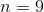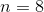Explanation:

To solve, isolate the variable by dividing both sides of your equation by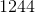: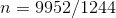As a check, know that any time you divide an even number by another even number, your result will be even.

Tired of practice problems?

Try live online GRE prep today.